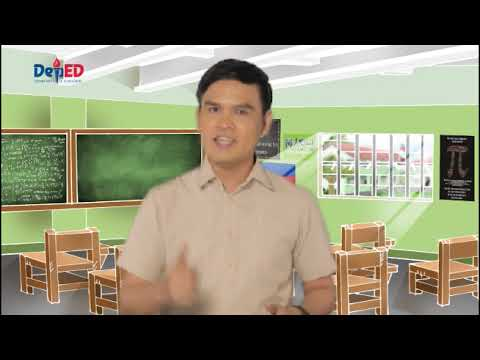### Grade 6 Math Q1 Ep1: Fractions

Grade 6 Math Quarter 1 Episode 1: Fractions Teacher: Marvin Olaso### Grade 6 Math Q1 Ep2: Subtracting Simple Fractions and Mixed numbers with and Without Regrouping

Grade 6 Math Quarter 1 Episode 2 : Subtracting Simple Fractions and Mixed numbers with and Without Regrouping Teacher : Marvin Olaso### Grade 6 Math Q1 Ep3: Solving Problems Involving Addition or Subtraction of Fractions

Grade 6 Math Quarter 1 Episode 3: Solving Problems Involving Addition or Subtraction of Fractions Teacher: Marvin Olaso### Grade 6 Math Q1 Ep4: Multiplying Simple Fractions and Mixed Fractions

Grade 6 Math Quarter 1 Episode 4 : Multiplying Simple Fractions and Mixed Fractions Teacher : Marvin Olaso### Grade 6 Math Q1 Ep5: Solving Routine and Non routine Problems Involving Multiplication

Grade 6 Math Quarter 1 Episode 5 : Solving Routine and Non routine Problems Involving Multiplication without or with Addition or Subtraction of Fractions and Mixed Fractions Teacher : Marvin Olaso### Grade 6 Math Q1 Ep6: Dividing Simple Fractions and Mixed Fractions

Grade 6 Math Quarter 1 Episode 6 : Dividing Simple Fractions and Mixed Fractions Teacher : Marvin Olaso### Grade 6 Math Q1 Ep7: Solving Routine or Non-routine Problems Involving Division of Fractions

Grade 6 Math Quarter 1 Episode 7: Solving Routine or Non-routine Problems Involving Division of Fractions Teacher: Marvin Olaso### Grade 6 Math Q1 Ep 8 Adding and Subtracting Decimals and Mixed Decimals through Ten Thousand

Grade 6 Math Quarter 1 episode 8 : Adding and Subtracting Decimals and Mixed Decimals through Ten Thousand Teacher : Marvin Olaso### Grade 6 Math Q1 Ep 9 Solving One or More Steps Problem Involving Add and/Or Sub of Decimals

Grade 6 Math Quarter 1 Episode 9 : Solving One or More Steps Problem Involving Addition and/Or Subtraction of Decimals Teacher : Marvin Olaso### Grade 6 Math Q1 Ep 10 Multiplying Decimals and Mixed Decimals Up to Two Decimal Places

Grade 6 Math Quarter 1 Episode 10 : Multiplying Decimals and Mixed Decimals Up to Two Decimal Places Teacher : Marvin Olaso### Grade 6 Math Q1 Ep 11 Solving Problem Involving Multiplication of Decimals

Grade 6 Math Quarter 1 Episode 11: Solving Problem Involving Multiplication of Decimals Teacher : Marvin Olaso### Grade 6 Math Q1 Ep 12 Solving Multi-Step Problems Involving Decimals

Grade 6 Math Quarter 1 Episode 12 : Solving Multi-Step Problems Involving Decimals Teacher : Marvin Olaso### Grade 6 Math Q1 Ep13: Dividing Whole Numbers by Decimals and Vice Versa

Grade 6 MATHEMATICS Quarter 1 Episode 13: Dividing Whole Numbers by Decimals and Vice Versa; Dividing Decimals or Mixed Decimals Teacher: Sir Marvin Olaso### Grade 6 Math Q1 Ep14: Dividing Decimals by 0.1, 0.01, 0.001, 10, 100, and 1000 Mentally

GRADE 6 Math Quarter 1 Episode 14: Dividing Decimals by 0.1, 0.01, 0.001, 10, 100, and 1000 Mentally Teacher: Marvin Olaso### Grade 6 Math Q1 Ep15: Differentiating Terminating from Repeating or Nonterminating Decimal Quotients

Grade 6 Math Quarter 1 Episode 15: Differentiating Terminating from Repeating or Nonterminating Decimal Quotients Teacher: Marvin Olaso### Grade 6 Math Q1 Ep16: Solving Multi-step Problems Involving Division of Decimals

Grade 6 Math Quarter 1 Episode 16: The Only Way to Learn Mathematics is to Live and Love Mathematics Teacher: Marvin Olaso